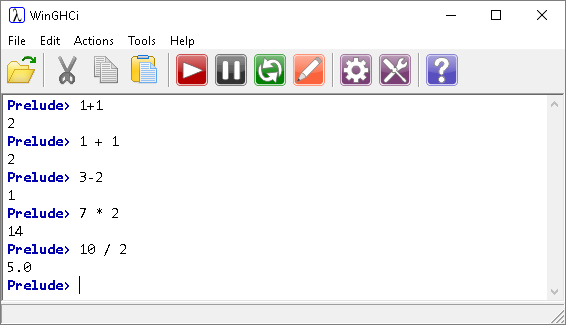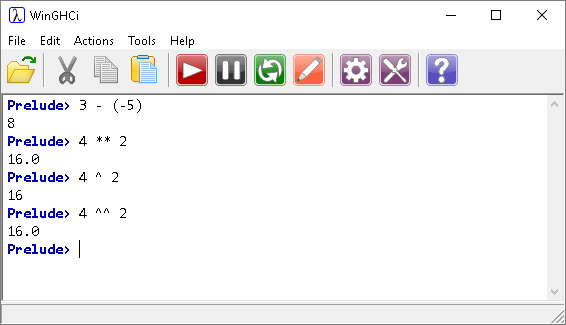# Introduction To Haskell Arithmetic Operators

## As You Might Expect

The four basic arithmetic operators are exactly what you would expect them to be,White space in Haskell is optional when using the operators.

To work with negative numbers, brackets must be put around the term or the compiler/interpreter has a hissy fit/gives an unexpected result. There are also some operators for powers.The decimal places in answers are hinting at the data type of the result.

A little bit of experimentation can show you that operator precedence is as you would expect,